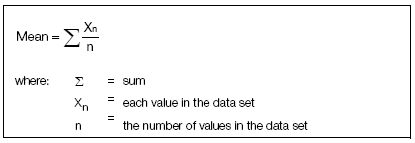##### Mean

The mean for a group of data points is simply the calculated average. The mean provides a laboratory's best estimate of the analyte's "true" value for a specific level of control. The mean ± a predetermined number of standard deviations represents the error expected in a test when the analytical system is stable. Use the following formula to calculate the mean: